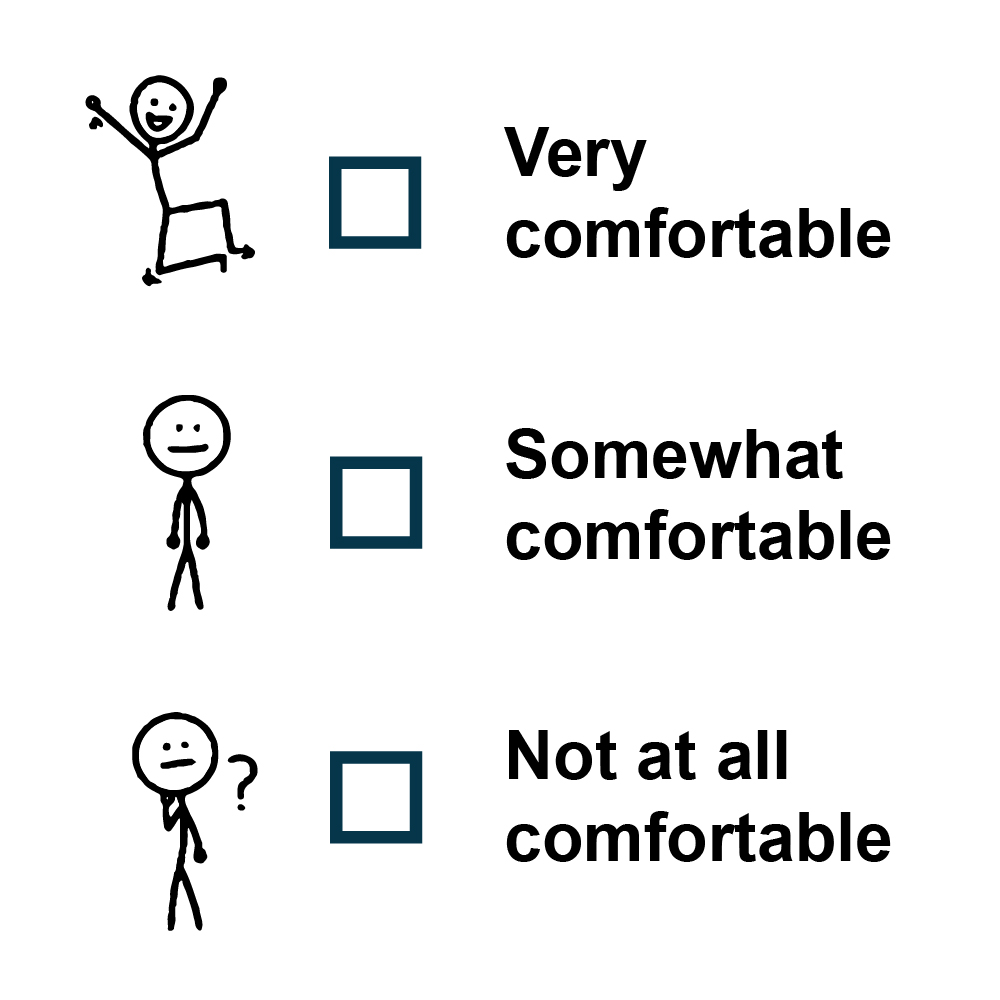Pages - Self-Assessment for Grade 9 Essentials Math (MAT1L)
 Home About Us      Board of Trustees 2018 - 2022 Board of Trustees Roles and Responsibilities Multi-Year Plan Board and Committee Meetings Public Participation Policies, Procedures & By-Laws Integrity Commissioner Schools        Programs & Services Students Parents Community
 You Are Here :

## Quick Launch

Self-Assessment for Grade 9 Essentials Math (MAT1L)

Students who are registered for Grade 9 Essentials Math (MAT1L) may benefit from a self evaluation and review of the followingexpectations from elementary school math.

The questions in this self-assessment reflect some of the key ideas learned in prerequisite courses.  They do not represent the problem solving approach or the rich experience that students would be exposed to in a classroom.  The intention is for students to revisit some key concepts and, if needed, access review materials in an informal environment at a pace that is comfortable for the student.

Concept(s)

Sample Question​​

How comfortable do you feel with this concept?

I can order whole numbers and decimal numbers

I can order fractional amounts with unlike denominators, including proper and improper fractions and mixed numbers

1. Write the following numbers in order from least to greatest:

1. 30     0.04     0.2     1.95     0.15

1.1.Comparing Decimals

Ordering Decimals

Different Denominators

Comparing Fractions

Mixed Numbers and Improper Fractions

I can print whole numbers in words

2. In 2019, there were 41, 205 secondary students in York Region District School Board.  Write out this number in words.Writing Numbers in Words

I can estimate quantities using benchmarks of 10%, 25%, 50%, 75%, and 100%

3. A student is walking from home to school.  If the student gets to the park, what percent of the distance has the student covered?Meaning of Percent

I can solve problems that arise from real-life situations

4. How many seconds are there in a day?How Many Seconds Are There In One Day?

I can add and subtract decimal numbers

I can multiply decimal numbers by whole numbers

I can divide decimal numbers by whole numbers

5. Evaluate:

1.1.1.Adding decimals with ones, tenths and hundredths

Introduction to multiplying decimals

Dividing a decimal by a whole number

I can represent ratios found in real-life contexts

6. A coin was flipped 20 times.  Heads came up 8 times.  What is the ratio of number of heads to number of tails?Introduction to ratios | Ratios, proportions, units, and rates

I can represent relationships using unit rates

7. Four markers cost \$5.16.  How much does one marker cost?Solving unit rates problem | Ratios, proportions, units, and rates

I can solve problems requiring conversion from larger to smaller metric units

8. A rug is 2.5 metres wide.  How many centimetres wide is it?Metric system: units of distance

# Solutions to sample​ questions

1. Write the following numbers in order from least to greatest:

a.  30     0.04     0.2     1.95     0.15

One way to sort these numbers is to start by writing them all with two digits after the decimal place.

30.00     0.04     0.20     1.95     0.15

Then we look at the place values to help us sort.

0.04     0.15     0.20     1.95     30.00

So the original list sorted is

0.04     0.15     0.2     1.95     30

b.One way to sort these numbers is to start by writing them all with two digits after the decimal place.

0.80          0.08        8.08          8.00

Then we look at the place values to help us sort.

0.08     0.80     8.00     8.08

So the original list sorted is

0.08     0.8     8    8.08

C.One way to sort this list is to write all of the fractions with the same denominator.  Note thatWe can now sort this listSo the original list in sorted order is2. In 2019, there were 41, 205 secondary students in York Region District School Board.  Write out this number in words.

There were forty one thousand two hundred and five secondary students in YRDSB.

3. A student is walking from home to school.  If the student gets to the park, what percent of the distance has the student covered?Since the student has walked 3 of the 4 parts and we know thatis 75%, the student has covered 75% of the distance.

4. How many seconds are there in a day?

There are 60 seconds in 1 minute and 60 minutes in an hour.  There are 24 hours in a day.  So the number of seconds in a day isseconds in a day.

5. Evaluate:

1.1.1.6. A coin was flipped 20 times.  Heads came up 8 times.  What is the ratio of number of heads to number of tails?

If there were 8 heads, then there were 12 tails.  The ratio is 8:12 or 2:3.

7. Four markers cost \$5.16.  How much does one marker cost?.  Each marker costs \$1.29

8. A rug is 2.5 metres wide.  How many centimetres wide is it?

1 metre is 100 centimetres so 2 meters is 200 centimetres.  Half a metre is 50 centimetres.  So 2.5 metres is 250 centimetres.